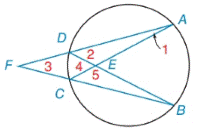Chapter 6.2, Problem 1EElementary Geometry For College St...

7th Edition
Alexander + 2 others
ISBN: 9781337614085

Solutions

Chapter
SectionElementary Geometry For College St...

7th Edition
Alexander + 2 others
ISBN: 9781337614085
Textbook Problem

Given: m A B ⌢ = 92 ° m D A ⌢ = 114 ° m B C ⌢ = 138 ° Find: a) m ∠ 1( ∠ D A C ) b) m ∠ 2 ( ∠ A D B ) c) m ∠ 3 ( ∠ A F B ) d) m ∠ 4 ( ∠ D E C ) e) m ∠ 5 ( ∠ C E B )To determine

(a)

To find:

m1 (DAC) when mAB=92°, mDA=114° and mBC=138°.

Explanation

Corollary:

The measure of an angle formed by a tangent and a chord drawn to the point of tangency is one-half the measure of the intercepted arc.

Calculation:

m1 (DAC).

mAB=92°, mDA=114° and mBC=138°.

By corollary,

m1=12(mCD)

mAB+mBC+mCD

To determine

(b)

To find:

m2 (ADB) when mAB=92°, mDA=114° and mBC=138°.

To determine

(c)

To find:

m3 (AFB) when mAB=92°, mDA=114° and mBC=138°

To determine

(d)

To find:

m4 (DEC) when mAB=92°, mDA=114° and mBC=138°.

To determine

(e)

To find:

m5 (CEB) when mAB=92°, mDA=114° and mBC=138°.

Still sussing out bartleby?

Check out a sample textbook solution.

See a sample solution

The Solution to Your Study Problems

Bartleby provides explanations to thousands of textbook problems written by our experts, many with advanced degrees!

Get Started

Evaluate the integrals in Problems 7-18. 12.

Mathematical Applications for the Management, Life, and Social Sciences

Using for |x| < 1 and differentiation, find a power series for .

Study Guide for Stewart's Multivariable Calculus, 8th

01e2xdx= a) 2e2 1 b) `e2 1 c) 12e2 d) 12(e21)

Study Guide for Stewart's Single Variable Calculus: Early Transcendentals, 8th